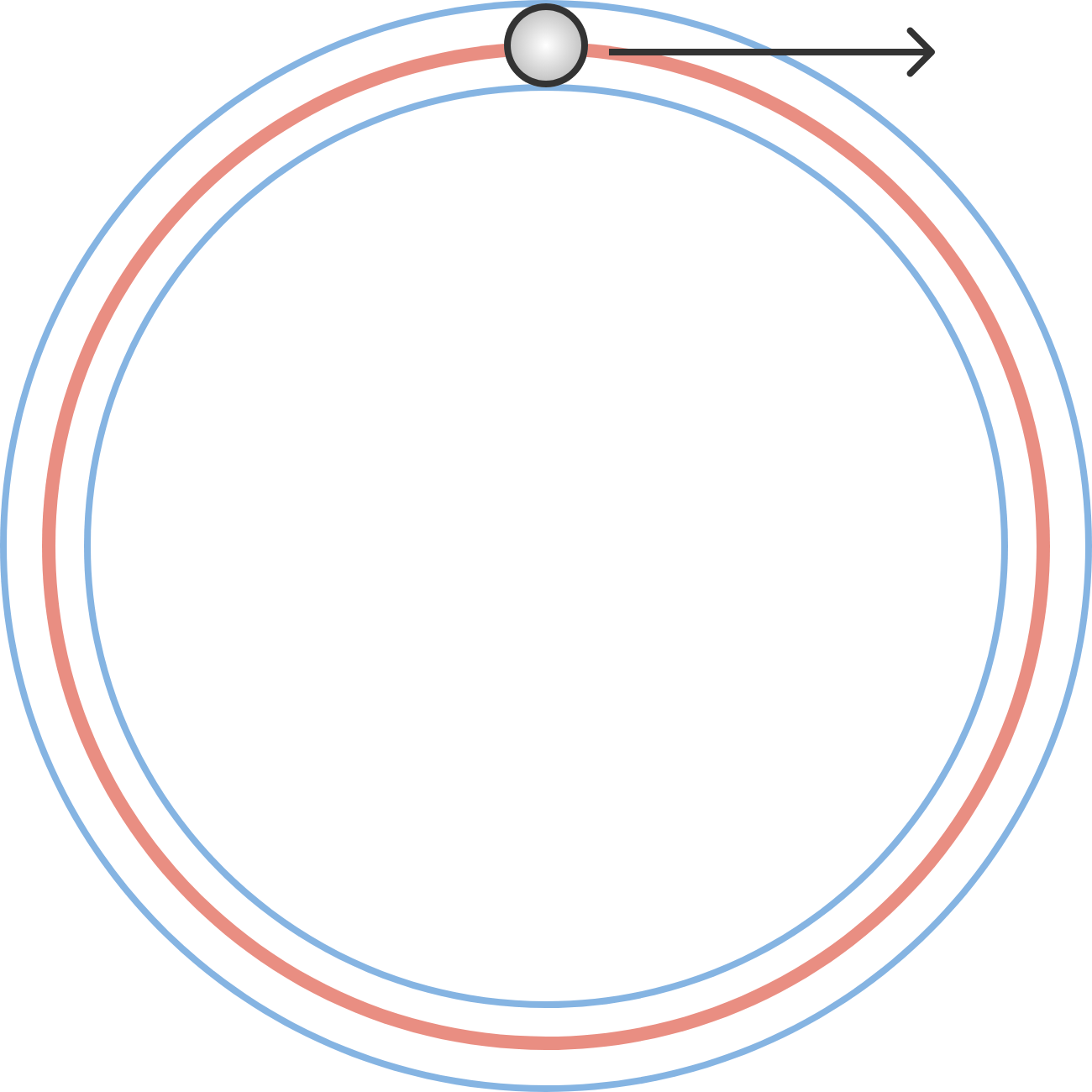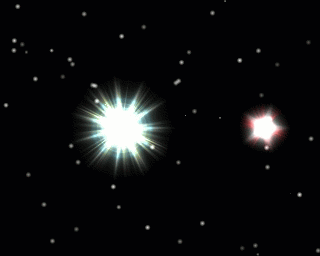# Problems of the Week

Contribute a problem

# 2017-06-12 Intermediate

You trace a circle (the red one) using a pen with a thick circular end. Both the outside and inside borders of the trace are also circles (in blue):If you trace any ellipse using the same kind of pen, will the outside and inside borders also be ellipses?Imagine two stars $A$ and $B$ forming a binary star system, where they revolve around a common point in space under their mutual gravitational attraction alone. If $A$ is heavier than $B$ and they both revolve in circular orbits, then which star will have a smaller orbital radius?

The first few powers of 101 all begin with 1, as highlighted by red colors below: \begin{aligned} 101^1 &=& {\color{#D61F06}{1}}01 \\ 101^2 &=& {\color{#D61F06}{1}}0201 \\ 101^3 &=& {\color{#D61F06}{1}}030301 \\ 101^4 &=& {\color{#D61F06}{1}}04060401 \\ &\vdots.& \end{aligned} Is that always the case for all powers of 101?

If $a + a^2 + a^3 + a^4 + a^5 + \cdots$ is a positive number, then which of the following is larger,

$a+a^3+a^5+a^7+\cdots\quad \text{or}\quad a^2+a^4+a^6+a^8+\cdots\, ?$

$\large \left ( 1 + \dfrac{1}{x} \right )^{x+1} = \left ( 1 + \dfrac{1}{1999} \right )^{1999}$

Find the sum of all real $x$ such that the above equation is true.

×